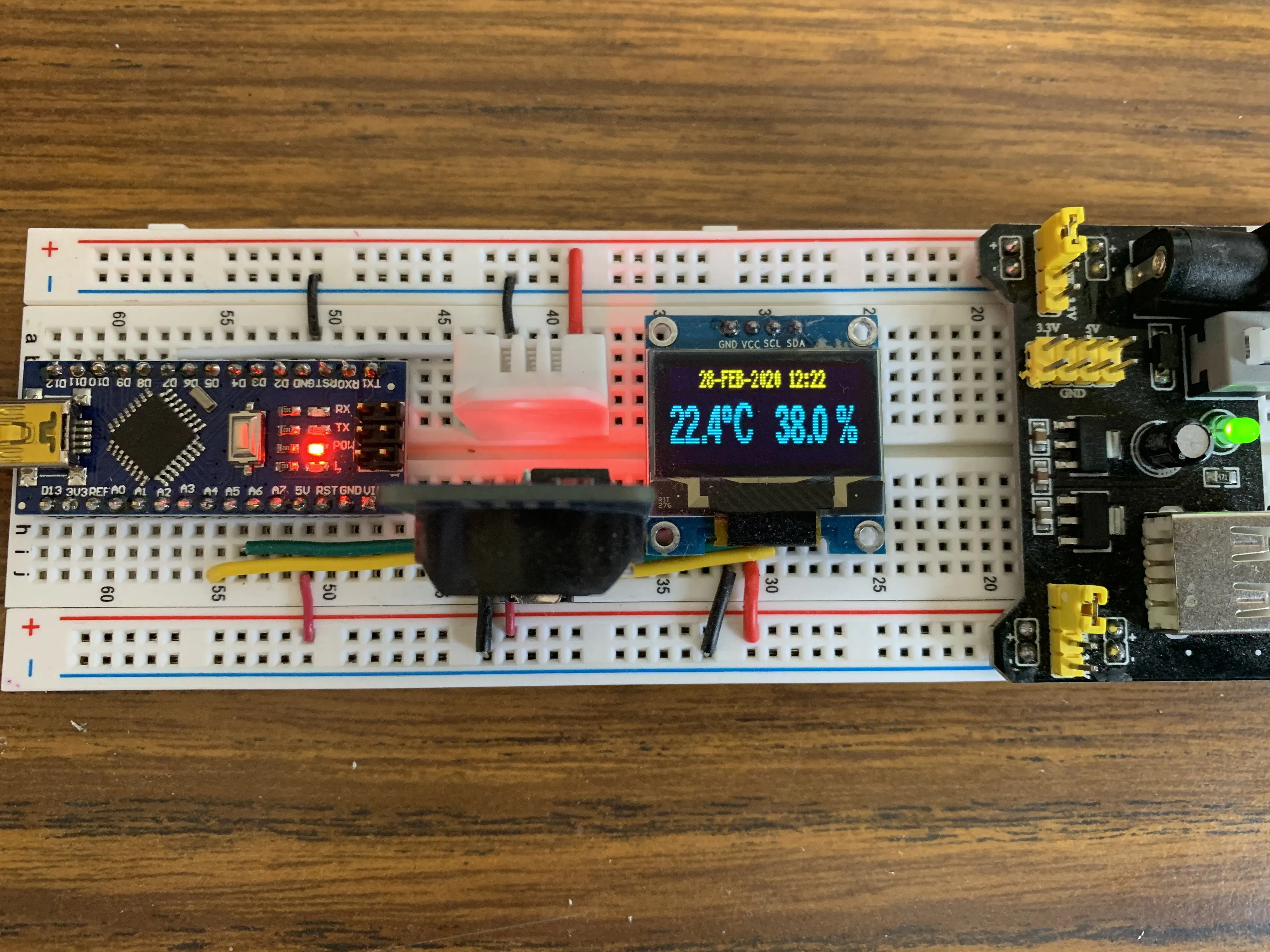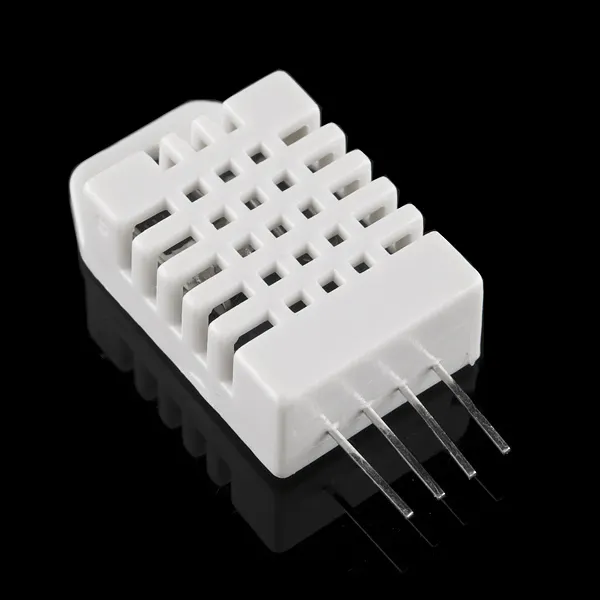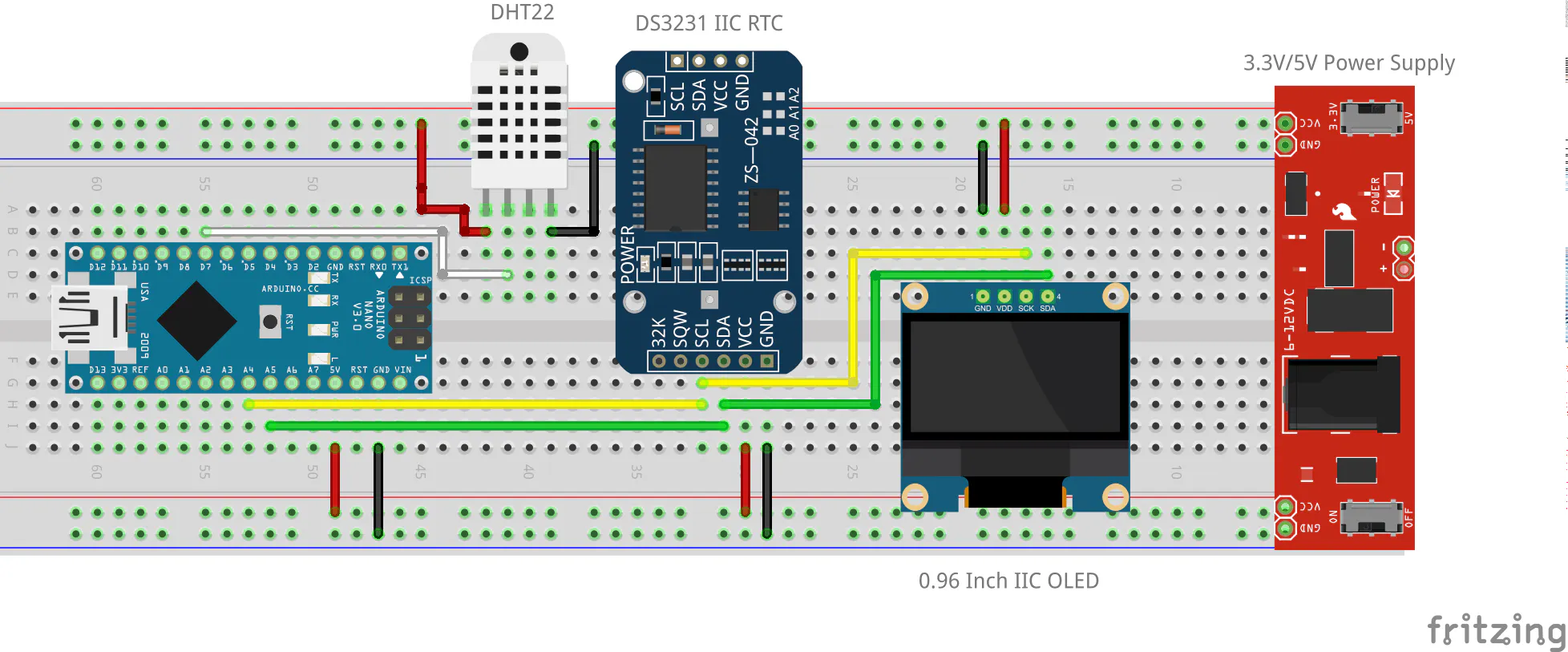# Arduino Nano Using Median Filter to Display DHT22 and RTC

Despike/smooth data from a DHT22 with a median filter and display on a 0.96 inch OLED with the date and time from a DS3231 real-time clock!

BeginnerFull instructions provided2,041## Things used in this project

### Hardware componentsDHT22 Temperature Sensor
×1
 Robojax DS3231 IIC Precision Real time Clock Module Any equivalent DS3231 IIC RTC should work
×1
 Kuman 0.96 Inch Yellow Blue IIC OLED Module I2c Any equivalent 0.96 inch OLED should work
×1Arduino Nano R3
×1

## Schematics## Code

### DHT Median filter with RTC

Arduino
```/*

Use an Arduino Nano to display temp/humidy on 0.96 inch Yellow/Blue IIC OLED I2c
serial 128x64 Display

Use an Adafruit DHT22 Temperature and Humidity Sensor (accuracy ±2%)

These data might bounce around somewhat because the sensor can be a little noisy
at times.  We use a simple median filter to smooth out the noise

Arduino Nano <--> OLED = pin A4 to SDA and pin A5 to SLC

February 2020

Paul M Dunphy, VE1DX

*/

// This library allows you to communicate with I2C devices, in this
// case the RTC and OLED display
#include <Wire.h>

// For the DS3231 Real time Clock Module (AT24C32 Module)
#include <DS1307RTC.h>

// Include DHT Library from Adafruit

#include "DHT.h";
/* DHT22 pin-out:
Pin 1 VCC
Pin 2 Data out
Pin 3 Not connected
Pin 4 Ground
*/

#include "U8glib.h"  // For the OLED - Obtain from: https://github.com/olikraus/u8glib

// Define Constants

#define dhtPin 7        // DHT-22 Output Pin connection
#define dhtType DHT22   // DHT Type is a DHT 22 (AM2302)

// Initialize OLED
U8GLIB_SSD1306_128X32 u8g(U8G_I2C_OPT_NONE);

// Initialize DHT sensor

DHT dht(dhtPin, dhtType);

tmElements_t tm;  // For the RTC

#define buff_size 7  // Must be an odd number.  Should be greater than 5.  7 works well.

unsigned long a_second = 1000;
float h = 0.0;  //Stores humidity value
float t = 0.0;  //Stores temperature value
float h_array[buff_size] = {0.0};
float t_array[buff_size] = {0.0};
float h_array_sort[buff_size] = {0.0};
float t_array_sort[buff_size] = {0.0};
String string_Temp, string_Humid, temporary_string, tick_string;

char OLED_string_1; // Need a couple of character buffers to hold the two OLED lines
char OLED_string_2;

String str_day, str_month, str_year, str_time; // Lot of conversion to strings required because the
// DSD Tech display doesn't like integers and floats

void clearOLED()
{
u8g.firstPage();
do {
} while( u8g.nextPage() );
}

void Initialize()
{
float startup_delay;
int i;

clearOLED();
startup_delay = buff_size * (a_second * 5.0 / 1000.0);
temporary_string  = String(startup_delay,0);
temporary_string = " (" + temporary_string + " seconds)";

tick_string = "Initializing ";
strcpy(OLED_string_1, tick_string.c_str());
strcpy(OLED_string_2, temporary_string.c_str());

// Take "buff_size" readings of each parameter, one every 5 seconds,
// to get initial arrays of data.  Print "status" dots across display.
for (i = 0 ; i < buff_size ; i++)
{
tick_string = tick_string + ". ";
strcpy(OLED_string_1, tick_string.c_str());
sendstringstoDisplay();
delay(a_second * 5);
h_array[i] = dht.readHumidity();       // Get Humidity value
t_array[i] = dht.readTemperature();    // Get Temperature value
}
}

void bubble_sort(float sort_array[], int n)
{
int i, j;
float  temp;

for (i = 0 ; i < n - 1; i++)
{
for (j = 0 ; j < n - i - 1; j++)
{
if (sort_array[j] > sort_array[j+1])
{
// Swap values
temp            = sort_array[j];
sort_array[j]   = sort_array[j+1];
sort_array[j+1] = temp;
}
}
}
}

void sendstringstoDisplay()
{
u8g.firstPage();  // Send them to the dispaly
do {

u8g.setFont(u8g_font_5x7);  // This can be adjusted to various fonts.  See:  https://github.com/olikraus/u8glib/wiki/fontsize
u8g.setPrintPos(0,7);       // Position of first line
u8g.print(OLED_string_1);
u8g.setFont(u8g_font_helvB14); // Little biger and bolder font as this is the temperature and humidy
u8g.setPrintPos(0, 25);        // Position of second line
u8g.print(OLED_string_2);

} while (u8g.nextPage() );
}

void monthStr(tmElements_t tm)
{

int int_month;

str_month = tm.Month;
int_month = str_month.toInt();

switch(int_month)
{
case 1:
str_month =("JAN");
break;
case 2:
str_month =("FEB");
break;
case 3:
str_month =("MAR");
break;
case 4:
str_month =("APR");
break;
case 5:
str_month =("MAY");
break;
case 6:
str_month =("JUN");
break;
case 7:
str_month =("JUL");
break;
case 8:
str_month =("AUG");
break;
case 9:
str_month =("SEP");
break;
case 10:
str_month =("OCT");
break;
case 11:
str_month =("NOV");
break;
case 12:
str_month =("DEC");
break;
default:
str_month =("ERR");
break;
}

}

void timeStr(tmElements_t tm)
{

// Add a leading zero when needed so all numbers are two characters

String hours,seconds,minutes;

if(tm.Hour<10)
{
hours = "0"+String(tm.Hour);
}
else
{
hours = String(tm.Hour);
}

if(tm.Minute<10)
{
minutes = "0"+String(tm.Minute);
}
else
{
minutes = String(tm.Minute);
}

// We're processing seconds "just in case", but not adding them to the small display

if(tm.Second<10)
{
seconds = "0"+String(tm.Second);
}
else
{
seconds = String(tm.Second);
}

str_time = hours + ":" + minutes;
}

void setup()
{
// Start Wire library for I2C
Wire.begin();
clearOLED();
dht.begin();
// initialize digital pin LED_BUILTIN as an output.
pinMode(LED_BUILTIN, OUTPUT);
Initialize();
}

void loop()
{
int i, median_index;

RTC.read(tm);  // Get the date and time
monthStr(tm);  // Convert the month from a number to Jan, Feb, Mar, etc
timeStr(tm);   // Convert the time to strings

h = dht.readHumidity();         // Get Humidity value
t = dht.readTemperature();      // Get Temperature value

// Replace the oldest value with the newest just read by moving every element in
// the arrays up one and sticking this new value in the bottom.
for (i = 0 ; i < buff_size - 1 ; i++)
{
h_array[i] = h_array[i + 1];
t_array[i] = t_array[i + 1];
}
h_array[buff_size-1] = h;
t_array[buff_size-1] = t;

// Move them into the sort arrays
for (i = 0 ; i < buff_size ; i++)
{
h_array_sort[i] = h_array[i];
t_array_sort[i] = t_array[i];
}

// Sort them. Use quick and dirty bubble sort because it's a small number of data points
bubble_sort(h_array_sort, buff_size);
bubble_sort(t_array_sort, buff_size);

// Use the median of the last "buff_zize" readings for the display
median_index = buff_size / 2;
h = h_array_sort[median_index];
t = t_array_sort[median_index];
string_Temp  = String(t,1);     // Make temp a character string
string_Humid = String(h,1);     // Make humid a character string

//  Build first OLED line
str_year = tmYearToCalendar(tm.Year);
str_day = tm.Day;
str_day = str_day + "-";
str_month = str_month + "-";
temporary_string = "    " + str_day + str_month + str_year + " " + str_time;
strcpy(OLED_string_1, temporary_string.c_str());      // Put date/time string in the first character array

//  Build second OLED line
temporary_string = "";
temporary_string = temporary_string + string_Temp + char(176) + "C   ";  // char(176) is the degree symbol
temporary_string = temporary_string + string_Humid + " %";
strcpy(OLED_string_2, temporary_string.c_str());      // Put temperature/humidity string in the second character array

sendstringstoDisplay();             // Display the median temperature and humidity
delay(5000);                        // Updates every 5 seconds

}
```

## Credits

### VE1DX

2 projects • 4 followers